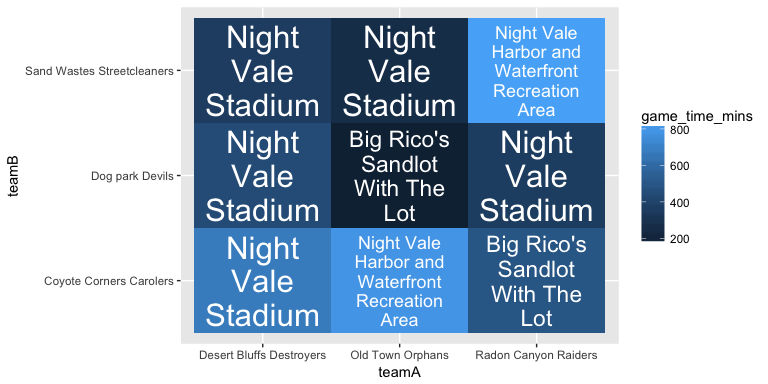‘ggfittext’ provides a ‘ggplot2’ geom for fitting text inside a box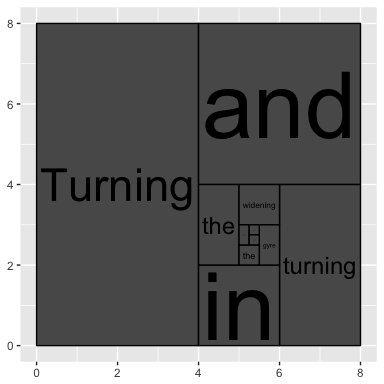# Installation

Install the release version of ‘ggfittext’ from CRAN:

``install.packages("ggfittext")``

If you want the development version, install it from GitHub:

``devtools::install_github("wilkox/ggfittext")``

# Fitting text inside a box

Sometimes you want to draw some text in a ‘ggplot2’ plot so that it fits inside a defined area. For example, you might want to label tiles in a heat map without letting the labels spill over into other tiles; or you might want to constrain some point labels to imaginary boxes so they don’t get too big. It’s possible to achieve this by manually fiddling with the text size, but this is both tedious and un-reproducible.

‘ggfittext’ provides a special geom called `geom_fit_text()` that automates fitting text inside a box. It works more or less like `ggplot2::geom_text()`, but provides some additional aesthetics and options that let you specify the box in which the text is to fit and how to make it fit.

``````library(ggplot2)
library(ggfittext)

flyers <- data.frame(
vehicle = rep(c("kite", "jumbo jet", "space shuttle", "dyson sphere"), 2),
xmin = rep(c(52.5, 45, 30, 0), 2),
xmax = rep(c(67.5, 75, 90, 120), 2),
ymin = rep(c(0, 15, 35, 60), 2),
ymax = rep(c(10, 30, 55, 85), 2),
geom = factor(c(rep("geom_text", 4), rep("geom_fit_text", 4)),
levels = c("geom_text", "geom_fit_text"))
)

ggplot(flyers, aes(label = vehicle, xmin = xmin, xmax = xmax, ymin = ymin,
ymax = ymax)) +
geom_rect() +
geom_text(data = subset(flyers, geom == "geom_text"),
aes(x = (xmin + xmax) / 2, y = (ymin + ymax) / 2)) +
geom_fit_text(data = subset(flyers, geom == "geom_fit_text")) +
facet_wrap( ~ geom) +
labs(x = "", y = "")``````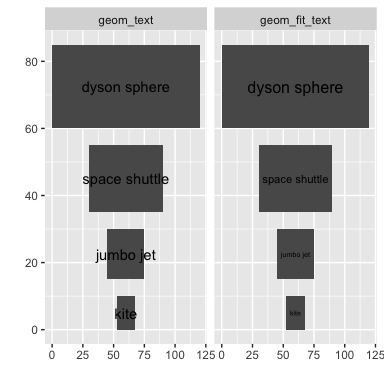You can define the width of the box with either ‘xmin’ and ‘xmax’ aesthetics, or alternatively with ‘x’ (for the horizontal centre of the box) and ‘width’ (in millimetres). Likewise, you can use either ‘ymin’ and ‘ymax’ or ‘y’ and ‘height’. The ‘x/width’ and ‘y/height’ aesthetics can be useful when using a discrete axis.

You can specify where in the box to place the text with the `place` argument, and a minimum point size for the text with the `min.size` argument. (Any text that would need to be smaller than `min.size` to fit the box will be hidden.)

``````ggplot(flyers, aes(label = vehicle, xmin = xmin, xmax = xmax, ymin = ymin,
ymax = ymax)) +
geom_rect() +
geom_text(data = subset(flyers, geom == "geom_text"),
aes(x = (xmin + xmax) / 2, y = (ymin + ymax) / 2)) +
geom_fit_text(data = subset(flyers, geom == "geom_fit_text"),
place = "top", min.size = 6) +
facet_wrap( ~ geom) +
labs(x = "", y = "")``````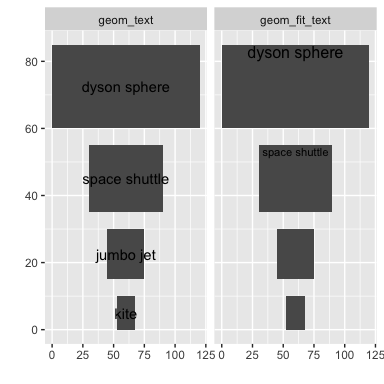Text can be placed in any corner (‘topleft’, ‘topright’, …) or at the midpoint of any side (‘bottom’, ‘left’, …), as well as the default ‘centre’.

# Growing text

With the `grow = TRUE` argument, text will be made to fill the box completely, whether that requires growing or shrinking it.

``````ggplot(flyers, aes(label = vehicle, xmin = xmin, xmax = xmax, ymin = ymin,
ymax = ymax)) +
geom_rect() +
geom_text(data = subset(flyers, geom == "geom_text"),
aes(x = (xmin + xmax) / 2, y = (ymin + ymax) / 2)) +
geom_fit_text(data = subset(flyers, geom == "geom_fit_text"), grow = T) +
facet_wrap( ~ geom, ncol = 1) +
labs(x = "", y = "")``````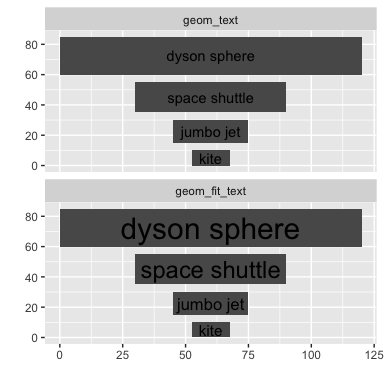# Reflowing text

With the `reflow = TRUE` argument, text will be reflowed (wrapped) as needed to fit the box. Reflowing is preferred to shrinking; that is, if the text can be made to fit by reflowing it without shrinking it, it will be reflowed only.

``````poem <- data.frame(
text = rep(
"Whose words these are I think I know.\nHe would prefer that they reflow",
3
),
xmin = rep(10, 3),
xmax = rep(90, 3),
ymin = rep(10, 3),
ymax = rep(90, 3),
fit = c("geom_text", "without reflow", "with reflow")
)

ggplot(poem, aes(xmin = xmin, xmax = xmax, ymin = ymin, ymax = ymax,
label = text)) +
geom_rect() +
geom_text(
data = subset(poem, fit == "geom_text"),
aes(x = (xmin + xmax) / 2, y = (ymin + ymax) / 2)
) +
geom_fit_text(data = subset(poem, fit == "without reflow"), min.size = 0) +
geom_fit_text(data = subset(poem, fit == "with reflow"), reflow = TRUE,
min.size = 0) +
lims(x = c(0, 100), y = c(0, 100)) +
labs(x = "", y = "") +
facet_wrap(~ fit)``````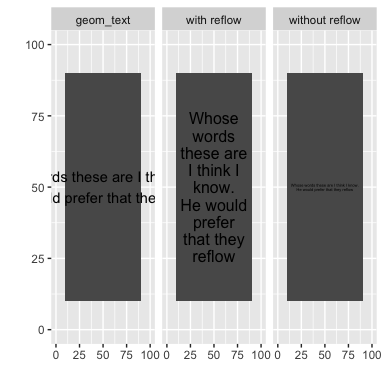Note that existing line breaks in the text are respected.

With both `reflow = TRUE` and `grow = TRUE`, the text will be reflowed to a form that best matches the aspect ratio of the box, then made to fill the box whether that requires shrinking it or growing it.

``````film <- data.frame(
text = rep("duck soup", 3),
xmin = rep(30, 3),
xmax = rep(70, 3),
ymin = rep(0, 3),
ymax = rep(100, 3),
fit = c("geom_text", "grow without reflow", "grow with reflow")
)

ggplot(film, aes(xmin = xmin, xmax = xmax, ymin = ymin, ymax = ymax,
label = text)) +
geom_rect() +
geom_text(
data = subset(film, fit == "geom_text"),
aes(x = (xmin + xmax) / 2, y = (ymin + ymax) / 2)
) +
geom_fit_text(data = subset(film, fit == "grow without reflow"), grow = TRUE) +
geom_fit_text(
data = subset(film, fit == "grow with reflow"),
grow = TRUE,
reflow = TRUE
) +
lims(x = c(0, 100), y = c(0, 100)) +
labs(x = "", y = "") +
facet_wrap(~ fit, ncol = 1)``````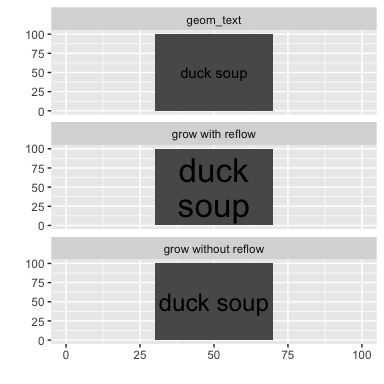# Example: a heatmap

``````tournament <- data.frame(
teamA = rep(c("Radon Canyon Raiders", "Desert Bluffs Destroyers",
"Old Town Orphans"), 3),
teamB = c(rep("Coyote Corners Carolers", 3),
rep("Sand Wastes Streetcleaners", 3),
rep("Dog park Devils", 3)),
venue = sample(c("Night Vale Stadium", "Big Rico's Sandlot With The Lot",
"Elementary School Playground",
"Night Vale Harbor and Waterfront Recreation Area"),
9, replace = T),
game_time_mins = sample(999, 9)
)

ggplot(tournament, aes(x = teamA, y = teamB, fill = game_time_mins,
label = venue)) +
geom_tile() +
geom_fit_text(width = 35, height = 25, min.size = 0, reflow = T, grow = T,
colour = "white")``````